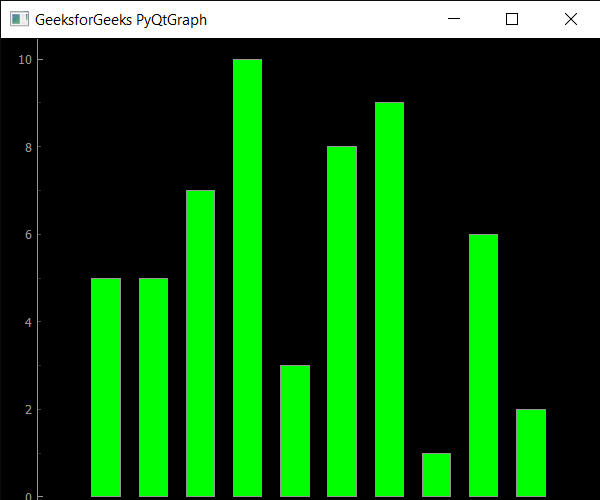PyQtGraph – Getting Maximum Height of Plot Window

• Last Updated : 24 Sep, 2020

In this article we will see how we can get the maximum height of the plot window in the PyQtGraph module. PyQtGraph is a graphics and user interface library for Python that provides functionality commonly required in designing and science applications. Its primary goals are to provide fast, interactive graphics for displaying data (plots, video, etc.) and second is to provide tools to aid in rapid application development (for example, property trees such as used in Qt Designer). Plot windows consist of two main parts: the Plot Panel containing the actual plotted graphics and the Control Panel. By default the plot window is resizable user can resize it any time, by setting maximum height we can fix that user will not be able to increase the height beyond the maximum height point. It can be set with the help of setMaximumHeight method.

We can create a plot window with the help of command given below

# creating a pyqtgraph plot window
window = pg.plot()

In order to do this we use maximumHeight method with the plot window object

Syntax : window.maximumHeight()

Argument : It takes no argument

Return : It returns integer

Below is the implementation

 # importing pyqtgraph as pgimport pyqtgraph as pg  # importing QtCore and QtGui from the pyqtgraph modulefrom pyqtgraph.Qt import QtCore, QtGui  # importing numpy as npimport numpy as np  import time  # creating a pyqtgraph plot windowwindow = pg.plot()  # setting window geometry# left = 100, top = 100# width = 600, height = 500window.setGeometry(100, 100, 600, 500)  # titletitle = "GeeksforGeeks PyQtGraph"  # setting window titlewindow.setWindowTitle(title)  # create list for y-axisy1 = [5, 5, 7, 10, 3, 8, 9, 1, 6, 2]  # create horizontal list i.e x-axisx = [1, 2, 3, 4, 5, 6, 7, 8, 9, 10]  # create pyqt5graph bar graph item # with width = 0.6# with bar colors = greenbargraph1 = pg.BarGraphItem(x = x, height = y1, width = 0.6, brush ='g')  # add item to plot window# adding bargraph item to the windowwindow.addItem(bargraph1)  # setting maximum height of the windowwindow.setMaximumHeight(600)  # getting maximum height of the windowvalue = window.maximumHeight()  # printing the valueprint("Max Height : ", end ="")print(value)    # main methodif __name__ == '__main__':          # importing system    import sys          # Start Qt event loop unless running in interactive mode or using     if (sys.flags.interactive != 1) or not hasattr(QtCore, 'PYQT_VERSION'):        QtGui.QApplication.instance().exec_()

Output :Max Height : 600

My Personal Notes arrow_drop_up